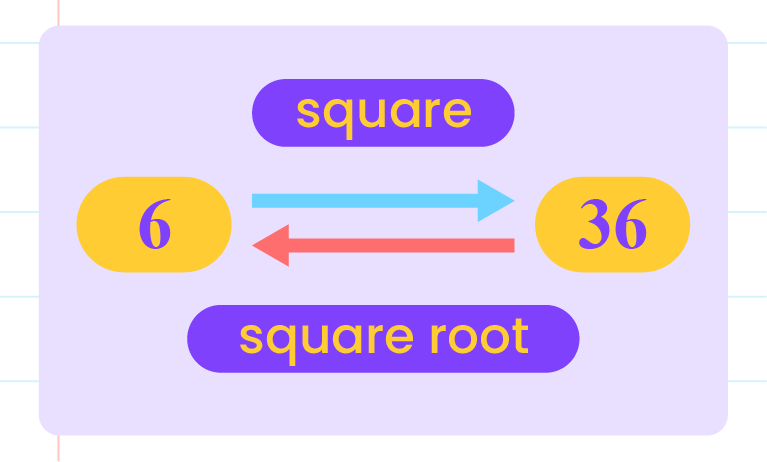## Squares and Square Roots

3.1  Squares and Square Roots

Squares:

 Definition A number that indicates a number is multiplied by the number itself.

• Examples: $$2^2,\, 9^2,\, 5^2$$

Perfect squares:

 Defintion A number that is equal to the square of a whole number.

• Examples: $$1,\, 4,\, 9$$

Determine a number is a perfect square:

• Perfect square can be written as a product of two equal factors.

 Example \begin{aligned} 144&=12\times12 \\\\&=12^2. \end{aligned} $$144$$ is a perfect square.

Relationship between squares and square roots:

• Finding the square and finding the square root are inverse operations.Example The square of $$8$$ is $$64$$. The square root of $$64$$ is $$8$$. $$8\times8=64$$ Thus, \begin{aligned} \sqrt{64}&=\sqrt{8\times8} \\\\&=8. \end{aligned}

The square of a number:

 Example Calculate: (i) \begin{aligned} 3^2&=3\times3 \\\\&=9. \end{aligned} (ii) \begin{aligned} \bigg(\dfrac{2}{5}\bigg)^2&=\dfrac{2}{5}\times\dfrac{2}{5} \\\\&=\dfrac{4}{25}. \end{aligned}

The square root of a number:

 Example Solve: (i) \begin{aligned} \sqrt{121}&=\sqrt{11\times11} \\\\&=\sqrt{11^2}\\\\&=11. \end{aligned} (ii) \begin{aligned} \sqrt{\dfrac{25}{49}}&=\sqrt{\dfrac{5}{7}\times\dfrac{5}{7}} \\\\&=\sqrt{\bigg(\dfrac{5}{7}\bigg)^2} \\\\&=\dfrac{5}{7}. \end{aligned}

Generalisation when two square roots are multiplied:

Square roots of the same numbers

• The product of two equal square root numbers is the number itself.
• $$\sqrt{a}\times\sqrt{a}=a$$

Square roots of different numbers

• The product of two different square root numbers is the square root of the product of the two numbers.
• $$\sqrt{a}\times\sqrt{b}=\sqrt{ab}$$